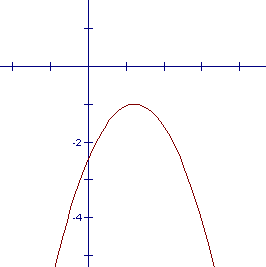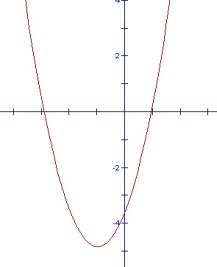#The Quadratic Formula

You may want to review preliminary information about quadratic functions and related concepts:

A quadratic equation is an equation of the form $\,ax^2 + bx + c = 0\,,$ where $\,a\ne 0\,.$

The quadratic formula solves quadratic equations. Just read off $\,a\,,$ $\,b\,,$ and $\,c\,,$ and substitute into the quadratic formula:

The Quadratic Formula: $$\cssId{s7}{x = \frac{-b\pm\sqrt{b^2-4ac}}{2a}}\ \ \text{(for a\ne 0)}$$

For example, solving the quadratic equation ‘$\,14x + 3x^2 = 5\,$’ is as simple as this:

$14x + 3x^2 = 5$
original equation
$\color{red}{3}x^2 + \color{blue}{14}x \color{green}{- 5} = 0$
Put the equation in standard form.
$\color{red}{a = 3}$
$\color{blue}{b = 14}$
$\color{green}{c = -5}$
After the equation is in standard form, read off:
• $a\,$ (the coefficient of the $\,x^2\,$ term)
• $b\,$ (the coefficient of the $\,x\,$ term)
• $c\,$ (the constant term)
Be sure to include the appropriate plus or minus signs!
\begin{align} \cssId{s21}{x}\ \ &\cssId{s22}{= \frac{-\color{blue}{14}\pm\sqrt{\color{blue}{14}^2 - 4\cdot \color{red}{3}\cdot (\color{green}{-5})}}{2\cdot \color{red}{3}}}\cr\cr &\cssId{s23}{= \frac{-14\pm\sqrt{256}}{6}}\cr\cr &\cssId{s24}{= \frac{-14\pm 16}{6}} \end{align}
Substitute into the quadratic formula and simplify: $$\cssId{s26}{x = \frac{-\color{blue}{b}\pm\sqrt{\color{blue}{b}^2 - 4\color{red}{a}\color{green}{c}}}{2\color{red}{a}}}$$
$\displaystyle \cssId{s27}{x} \cssId{s28}{= \frac{-14 \,\color{orange}{\large{\mathbf{+}}} \,16}{6}} \cssId{s29}{= \frac{2}{6}} \cssId{s30}{= \frac 13}$

or

$\displaystyle \cssId{s32}{x} \cssId{s33}{= \frac{-14\,\color{red}{\large{\mathbf{-}}}\,16}{6}} \cssId{s34}{= \frac{-30}{6}} \cssId{s35}{= -5}$
The symbol ‘$\,\pm\,$’ means ‘plus or minus’.

Write the ‘$\,\color{orange}{\large{\mathbf{+}}}\,$’ and ‘$\,\color{red}{\large{\mathbf{-}}}\,$’ solutions separately.

$\begin{gather} \cssId{s40}{14(\frac 13) + 3\bigl(\frac 13\bigr)^2 \overset{?}{=} 5}\cr\cr \cssId{s41}{\frac{14}{3} + \frac{1}{3} \overset{?}{=} 5}\cr\cr \cssId{s42}{\frac{15}{3} \overset{?}{=} 5}\cr\cr \cssId{s43}{5 = 5}\cr\cr\cr \cssId{s44}{14(-5) + 3\bigl(-5)^2 \overset{?}{=} 5}\cr\cr \cssId{s45}{-70 + 75 \overset{?}{=} 5}\cr\cr \cssId{s46}{5 = 5} \end{gather}$
If you've got time, check your solutions in the original equation. Don't drop the question mark over the equal sign until you're sure they're equal. Voila!

Quadratic functions are functions that can be written in the form $\,ax^2 + bx + c\,$ for $\,a\ne 0\,.$ Every quadratic function graphs as a parabola with directrix parallel to the $x$-axis.

The graphs of quadratic functions can have three different $x$-intercept situations, as shown below:

• no $x$-intercepts
• exactly one $x$-intercept
• two different $x$-interceptsno $x$-interceptsexactly one $x$-intercepttwo different $x$-intercepts

Points on the $x$-axis have their $y$-value equal to zero. Thus, to find the $x$-intercepts for any curve, you set $\,y\,$ equal to zero and solve for $\,x\,.$

In particular, to find the $x$-intercepts of a quadratic function $\,y = ax^2 + bx + c\,$  ($\,a\ne 0\,$), it is necessary to solve the equation $\,ax^2 + bx + c = 0\,$ (which is called a quadratic equation).

The formula that gives the solutions to this equation is called the quadratic formula, and is derived next.

## Derivation of the Quadratic Formula

### Solving the Equation $\,ax^2 + bx + c = 0\,,$ where $\,a\ne 0\,$

Using the technique of completing the square, the equation is transformed to the form $\,z^2 = k\,,$ as follows:

$ax^2 + bx + c = 0$
original equation
$ax^2 + bx = -c$
Subtract $\,c\,$ from both sides.
$\displaystyle x^2 + \frac{b}{a}x = -\frac{c}{a}$
Divide both sides by $\,a\ne 0\,$; the technique of completing the square only works when the coefficient of the squared term is $\,1\,.$
$\displaystyle x^2 + \frac{b}{a}x + {(\frac{b}{2a})}^2 = -\frac{c}{a} + \frac{b^2}{4a^2}$
Notice that: $$\cssId{s79}{\frac{b/a}{2}} \cssId{s80}{= \frac{b}{a}\div 2} \cssId{s81}{= \frac{b}{a}\cdot \frac{1}{2}} \cssId{s82}{= \frac{b}{2a}}$$ Add $\,{(\frac{b}{2a})}^2 = \frac{b^2}{4a^2}\,$ to both sides; this is the correct number to add to turn the left side into a perfect square.
$\displaystyle {\left(x + \frac{b}{2a}\right)}^2 = -\frac{c}{a}\cdot \frac{4a}{4a} + \frac{b^2}{4a^2}$
Rewrite left side as a perfect square; get a common denominator on the right.
$\displaystyle {\left(x + \frac{b}{2a}\right)}^2 = \frac{b^2 - 4ac}{4a^2}$
$\displaystyle x + \frac{b}{2a} = \pm\sqrt{\frac{b^2 - 4ac}{4a^2}}$
The equation $\,z^2 = k\,$ has solutions $\,z=\pm\sqrt{k}\ .$
Recall that $\,z=\pm\sqrt{k}\,$ is just a convenient shorthand for:
$$z = \sqrt{k}\ \ \text{or}\ \ z = -\sqrt{k}$$
$\displaystyle x + \frac{b}{2a} = \pm \frac{\sqrt{b^2-4ac}}{\sqrt{4a^2}}$
Use the rule: $$\sqrt{\frac{A\strut}{B\strut}} = \frac{\sqrt{A}}{\sqrt{B}}$$
$\displaystyle x + \frac{b}{2a} = \pm \frac{\sqrt{b^2-4ac}}{\sqrt{4}\sqrt{a^2}}$
Use the rule: $$\sqrt{AB\strut} = \sqrt{A\strut}\cdot\sqrt{B\strut}$$
$\displaystyle x + \frac{b}{2a} = \pm \frac{\sqrt{b^2-4ac}}{2|a|}$
Use the rule: $$\sqrt{A^2\strut} = |A|\,$$
Notice that if $\,a\gt 0\,,$ then $\,|a|=a\,,$
so the right side of the previous equation becomes: $$\pm\frac{\sqrt{b^2-4ac}}{2a}$$
Also, if $\,a\lt 0\,,$ then $\,|a|=-a\,,$
so the right side becomes: $$\pm\frac{\sqrt{b^2-4ac}}{2(-a)} = \mp\frac{\sqrt{b^2-4ac}}{2a}$$

In both cases, the same two values for the right side result. Thus, we continue with a simplified right side:

$\displaystyle x + \frac{b}{2a} = \pm \frac{\sqrt{b^2-4ac}}{2a}$
The plus/minus allows elimination of the absolute value.
$\displaystyle x=-\frac{b}{2a} \pm \frac{\sqrt{b^2-4ac}}{2a}$
Add $\,-\frac{b}{2a}\,$ to both sides.
$\displaystyle x=\frac{-b\pm\sqrt{b^2-4ac}}{2a}$

In summary, we have:

Let $\,a\ne 0\,.$

The solutions to the equation $\,ax^2 + bx + c = 0\,$ are given by:

$$\cssId{s117}{x = \frac{-b\pm\sqrt{b^2-4ac}}{2a}}$$

The expression $\,b^2 - 4ac\,$ that appears under the square root in the quadratic formula is critical in determining the nature of the solutions to the quadratic equation $\,ax^2 + bx + c = 0\,,$ as follows:

### Positive Discriminant

If $\,b^2 - 4ac\gt 0\,,$ then $\sqrt{b^2-4ac}\,$ is a positive real number.

In this case, $$\cssId{s123}{\frac{-b \color{red}{+ \sqrt{b^2-4ac}}}{2a}}$$ and $$\cssId{s124}{\frac{-b \color{red}{- \sqrt{b^2-4ac}}}{2a}}$$ are different real numbers. Thus, there are two different real number solutions to the quadratic equation $\,ax^2 + bx + c = 0\,,$ and the graph of the quadratic function $\,ax^2+bx+c\,$ has two different $x$-intercepts.

### Discriminant Equal to Zero

If $\,b^2 - 4ac= 0\,,$ then $\sqrt{b^2-4ac} = 0\,.$

In this case, $$\cssId{s131}{\frac{-b + \sqrt{b^2-4ac}}{2a} = \frac{-b\color{red}{+0}}{2a}}$$ and $$\cssId{s132}{\frac{-b - \sqrt{b^2-4ac}}{2a} = \frac{-b\color{red}{-0}}{2a}}$$ are the same numbers.

Thus, there is exactly one real number solution to the quadratic equation $\,ax^2 + bx + c = 0\,,$ and the graph of the quadratic function $\,ax^2 + bx + c\,$ has only one $x$-intercept.

### Negative Discriminant

If $\,b^2 - 4ac\lt 0\,,$ then $\,\sqrt{b^2-4ac}\,$ is not a real number.

In this case, there are no real number solutions to the quadratic equation $\,ax^2 + bx + c = 0\,,$ and the graph of the quadratic function $\,ax^2+bx+c\,$ has no $x$-intercepts.

### The Discriminant Discriminates...

Thus, the expression $\,b^2 - 4ac\,$ helps us to discriminate between the various types of solutions to a quadratic equation, and the various $x$-intercept situations for a quadratic function.

Thus, we have the following definition:

DEFINITION discriminant

Let $\,a\ne 0\,.$

The expression $\,b^2 - 4ac\,$ is called the discriminant of the quadratic equation $\,ax^2 + bx + c = 0\,.$

Similarly, the expression $\,b^2 - 4ac\,$ is called the discriminant of the quadratic function $\,ax^2 + bx + c\,.$